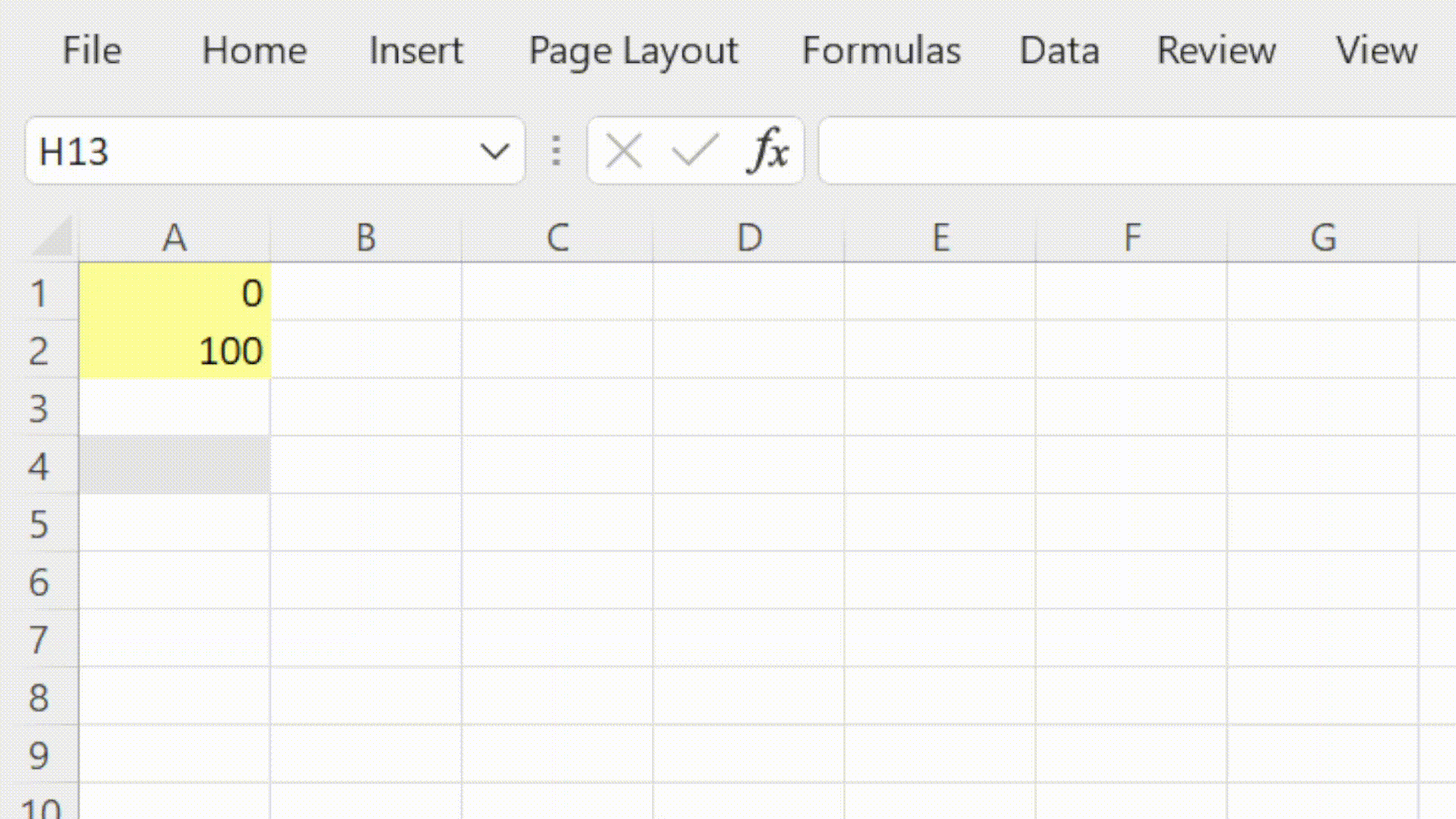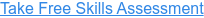Back to Blog# How to use Excel's MIN Function

Published
Jun 18, 2021 11:01:00 AM

Returns the smallest value from a range of numbers

### What does Excel's MIN function do?

The MIN Function is one of Excel's Statistical functions.

The MIN function returns the smallest value in the range of numbers provided.

In financial modelling the MIN function is very useful for ensuring that the result of a calculation does not go above a given threshold.

In cashflow forecasting we can use the MIN function to show the lowest closing cash balance.

### Here's a simple example

Let's take a look at a simple example of the MIN function.

We would like to know the smallest number in cells A1 and A2 (or that range A1 to A2).

#### =MIN(A1, A2)  or  =MIN(A1:A2)### What does that mean in plain English?

Return the smallest number from the values stored in cells A1 and A2 (or in the range A1 to A2).

### How do I write a formula using the MIN function?

#### What to consider when using the MIN function in your financial model

• Values can be hardcoded, selected singularly (up to 255) or as ranges.
• MIN ignores empty cells, text values and TRUE & FALSE.
• Use the MINA function if you would like to include empty cells, text values and TRUE & FALSE

Read more about the MIN function on the Microsoft support page here.

#### Take our free 30-question skills assessment

See where you stand with your Excel, financial modelling, data visualisation & analytics, and modelling tech skills